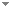Switch to: References

# Brouwer's fan theorem and unique existence in constructive analysis

Mathematical Logic Quarterly 51 (4):360-364 (2005)

 Continuous Homomorphisms of R Onto a Compact Group.Douglas Bridges & Matthew Hendtlass - 2010 - Mathematical Logic Quarterly 56 (2):191-197.It is shown within Bishop's constructive mathematics that, under one extra, classically automatic, hypothesis, a continuous homomorphism from R onto a compact metric abelian group is periodic, but that the existence of the minimum value of the period is not derivable. Direct download     Export citationBookmarkConstructing Local Optima on a Compact Interval.Douglas S. Bridges - 2007 - Archive for Mathematical Logic 46 (2):149-154.The existence of either a maximum or a minimum for a uniformly continuous mapping f of a compact interval into ${\mathbb{R}}$ is established constructively under the hypotheses that f′ is sequentially continuous and f has at most one critical point. Direct download (3 more)     Export citationBookmark1 citation The Binary Expansion and the Intermediate Value Theorem in Constructive Reverse Mathematics.Josef Berger, Hajime Ishihara, Takayuki Kihara & Takako Nemoto - 2019 - Archive for Mathematical Logic 58 (1-2):203-217.We introduce the notion of a convex tree. We show that the binary expansion for real numbers in the unit interval ) is equivalent to weak König lemma ) for trees having at most two nodes at each level, and we prove that the intermediate value theorem is equivalent to \ for convex trees, in the framework of constructive reverse mathematics. No categories Direct download (2 more)     Export citationBookmarkToward a Clarity of the Extreme Value Theorem.Karin U. Katz, Mikhail G. Katz & Taras Kudryk - 2014 - Logica Universalis 8 (2):193-214.We apply a framework developed by C. S. Peirce to analyze the concept of clarity, so as to examine a pair of rival mathematical approaches to a typical result in analysis. Namely, we compare an intuitionist and an infinitesimal approaches to the extreme value theorem. We argue that a given pre-mathematical phenomenon may have several aspects that are not necessarily captured by a single formalisation, pointing to a complementarity rather than a rivalry of the approaches. Direct download (6 more)     Export citationBookmark2 citations Brouwer’s Fan Theorem as an Axiom and as a Contrast to Kleene’s Alternative.Wim Veldman - 2014 - Archive for Mathematical Logic 53 (5-6):621-693.The paper is a contribution to intuitionistic reverse mathematics. We introduce a formal system called Basic Intuitionistic MathematicsBIM, and then search for statements that are, over BIM, equivalent to Brouwer’s Fan Theorem or to its positive denial, Kleene’s Alternative to the Fan Theorem. The Fan Theorem is true under the intended intuitionistic interpretation and Kleene’s Alternative is true in the model of BIM consisting of the Turing-computable functions. The task of finding equivalents of Kleene’s Alternative is, intuitionistically, a nontrivial extension (...) Direct download (8 more)     Export citationBookmark8 citations Some Axioms for Constructive Analysis.Joan Rand Moschovakis & Garyfallia Vafeiadou - 2012 - Archive for Mathematical Logic 51 (5-6):443-459.This note explores the common core of constructive, intuitionistic, recursive and classical analysis from an axiomatic standpoint. In addition to clarifying the relation between Kleene’s and Troelstra’s minimal formal theories of numbers and number-theoretic sequences, we propose some modified choice principles and other function existence axioms which may be of use in reverse constructive analysis. Specifically, we consider the function comprehension principles assumed by the two minimal theories EL and M, introduce an axiom schema CFd asserting that every decidable property (...) Direct download (6 more)     Export citationBookmark1 citation Convexity and Unique Minimum Points.Josef Berger & Gregor Svindland - 2019 - Archive for Mathematical Logic 58 (1-2):27-34.We show constructively that every quasi-convex, uniformly continuous function \ with at most one minimum point has a minimum point, where C is a convex compact subset of a finite dimensional normed space. Applications include a result on strictly quasi-convex functions, a supporting hyperplane theorem, and a short proof of the constructive fundamental theorem of approximation theory. No categories Direct download (2 more)     Export citationBookmark1 citation Brouwer’s Fan Theorem and Convexity.Josef Berger & Gregor Svindland - 2018 - Journal of Symbolic Logic 83 (4):1363-1375. Direct download (2 more)     Export citationBookmark